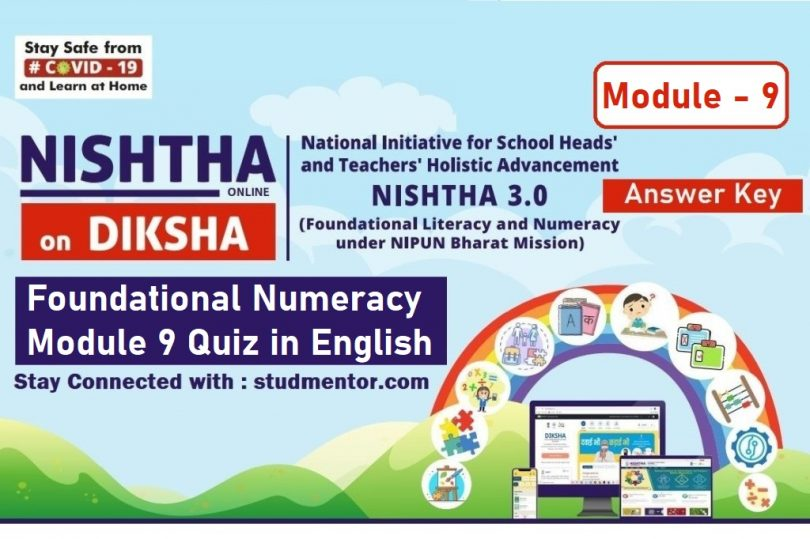# Nishtha 3.0 Diksha Portal Module 9 Quiz 2022 Answer KeyWritten by

1. Which of the following is not a correct way of assessment

Answer – A test based on memorisation

2. ____________Numbers are used to communicate the size of a group of objects.

3. Which of the following pre-school teachers should avoid?

4. The ability to immediately perceive the cardinality of a collection, usually not more than four or five elements without counting is called as

5. Essential requirements to classify objects is to:

Answer – Identify the objects by their characteristics

6. What are Numerals ?

7. The concept of ‘zero’ can be introduced best through which of the following operations?

8. During the process of counting, a child doesn’t

9. What is subitising ?

Answer – Ability to identify the number of objects by simply looking at them and without actually counting each objects.

10. Which of the following is not a component of foundational numeracy:

11. In order to ensure strong FLN the children should be assessed –

12. Which of the following is not a pre number skill:

13. How many times should we add 4 to get 16

14. Which of the following is not a mathematical process?

15. Which of the following pairs are not complementary to each other ?

16. Which of the following is not true:

Answer – All rectangles are squares

17. Which of the following is not a dimension of assessments of mathematics learning?

18. child should be able to seriate objects before learning numbers, because seriation is:

Answer – related with ordination or placing numbers in order

19. During the learning of Mathematics at early stages, a child is not expected to-

Answer – Learn conventions needed for Mathematical techniques

20. Which of the following is not a key skill to develop under Number sense

Answer – Recitation of number names

## Example 2 (Means Second Time I Tried Quiz so All Questions are Involve)

1. Which of the following is the most appropriate strategy to teach shapes at a foundational stage?

Answer – Children should be given ample opportunities to develop intuitive understanding of shapes

2. The process by which information is exchanged between individuals through mathematical symbols, signs, diagrams, graphs is known as

3. Which of the following does not involve the ordering a collection of objects according to the given rule.

4. Putting together things that have some characteristics in common enhances the competence of

5. Which of the following scenarios is not involved in the word problems related to addition and Subtraction?

6. Activities on matching or pairing of objects will help in the development of which pre-number skill

Answer – One to one correspondence

7. When does a child is said not to acquire understanding of shapes and space ?

Answer – When he/she crams the names of shapes like cube, cuboid, sphere, etc. without understanding

8. ___________Number are used to describe the position of an object when they are arranged in a specific order.

9. Which of the following is not a pedagogical process to enhance foundational Numeracy skill:

Answer – Giving lots of practice questions

10. ‘Seema has 12 roses. Shifa has 15 roses. Who has more and by how much?’ What subtraction context has been used in the above word problem?

11. Which of the following is not an objective of making a child proficient in numeracy in the foundational years?

Answer – It helps them to do fast calculations

12. For building upon the understanding of one-to-one correspondence, children do not need to understand the meaning of

13. Which of the following activities is best suited for the development of spatial understanding among children?

Answer – Drawing the front view of a bottle

14. Which of the following is the most crucial aspect of learning multiplication ?

Answer – Understanding multiplication as finding “how many times”

15. Which of the following should not be an approach for teaching measurement ?

Answer :- Directly introducing standard units of measurements by the teacher and their conversations

16. Which of the following is not a component of Data Handling?

17. Which of the following is not a type and utility of numbers;

18. What should be the appropriate sequence in learning/understanding multiplication?

• I. Applying distributive law of multiplication w.r.t addition
• II. Understanding the meaning of multiplication
• III. Learning the algorithm of multiplication
• IV. Understanding and using the language of multiplication

19. Which of the following does not involve one to one correspondence ?

20. What is the right sequence to teach numbers:

1. Opportunities for counting
2. Writing numerals
4. Developing number sense

## Read Also Previous Nishtha 3.0 Modules

I Hope you like the Article of the Nishtha 3.0 Diksha Portal Module 9 Quiz 2022 Answer Key. if you like then share to others.